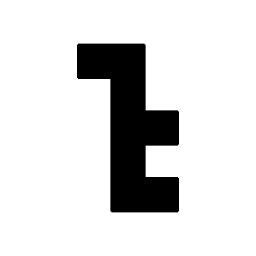## Tuesday, September 9, 2014

### Fun with Bordered Cubes

I am interested in generating 3D reasoning items in R. To this end I have adapted some of the awesome functions built in the rgl library to my ends. My new function is 'cube' and it takes position and automatically sizes itself as a 1x1x1 cube though this can be adjusted through the scale argument.

`Find the code on github `
` `
```# Easy Bordered Cubes in R

library('rgl'); library('magrittr')

cube <- function(x=0,y=0,z=0, bordered=TRUE,
filled = TRUE, lwd=2, scale=1,
fillcol = gray(.95),
bordercol ='black', ...) {

mycube <- cube3d()

# Reduce size to unit
mycube\$vb[4,] <- mycube\$vb[4,]/scale*2

for (i in 1:length(x)) {
if (bordered) {
bcube <- mycube
bcube\$material\$lwd <- lwd
bcube\$material\$front <- 'line'
bcube\$material\$back <- 'line'
bcube %>% translate3d(x[i], y[i], z[i]) %>% shade3d
}
if (filled) {
fcube <- mycube
fcube\$vb[4,] <- fcube\$vb[4,]*1.01
fcube\$material\$col <- fillcol
fcube %>% translate3d(x[i], y[i], z[i]) %>% shade3d
}
}
}

clear3d()
cube(0,0,0)
cube(1,0,0, filled=F)
cube(-1,0,0, bordered=F)
movie3d(spin3d(axis=c(0,0,1), rpm=20), duration=2.95) ```
```
# I mapped R using an excel spreadsheet which
# translated Xs into 2D locations points
clear3d()
y <- c(1,1,1,1,1,1,2,2,2,2,3,3,3,3,4,4,4,4,4,5,5,
5,5,6,6,6,6,7,7,7,7,7,8,8,8,8,9,9,9,9,10,
10,10,10,11,11,11,11,11,12,12,12,12,12)

x <- c(8,7,6,3,2,1,7,6,3,2,6,5,3,2,6,5,4,3,2,5,4,
3,2,5,4,3,2,6,5,4,3,2,7,6,3,2,7,6,3,2,7,6,
3,2,6,5,4,3,2,5,4,3,2,1)

z <- cummax(y)*.5

length(x)==length(y)
cube(x,y,z)
movie3d(spin3d(axis=c(0,0,1), rpm=20), duration=2.95) ```
` `
``` # Let's see how sin and cos can work together
z <- seq(0,6,.1)
x <- sin(pi*z)*z
y <- cos(pi*z)*z

clear3d()
cube(x,y,z*2, scale=.75)
movie3d(spin3d(axis=c(0,0,1), rpm=20), duration=2.95) ```
` `
` `
```# Now let's look at some of the prototyping for
# my 3D reasoning items.
clear3d()
vlist <- c(0,0,0)
for (i in 1:15) {
cube(vlist,vlist,vlist)
step <- sample(1:3, 1)
vlist[step] <- vlist[step]+(-1)^rbinom(1,1,.25)
}
rgl.material(shininess=1)
bg3d("white")
clear3d(type = "lights")

rgl.viewpoint(theta = 90, phi = 0, fov = 0)
rgl.snapshot("2014-09-09angle1.png") ```
` `
` `
```rgl.viewpoint(theta = 0, phi = 0, fov = 0)
rgl.snapshot("2014-09-09angle2.png") ```
` `
` `
```rgl.viewpoint(theta = 0, phi = 90, fov = 0)
rgl.snapshot("2014-09-09angle3.png")rgl.light()
rgl.viewpoint(theta = 180, phi = 20, fov = 60)
rgl.snapshot("2014-09-09cubes3d1.png") ```
` `
` `
```rgl.viewpoint(theta = -20, phi = -20, fov = 60)
rgl.snapshot("2014-09-09cubes3d2.png")```
` `
` `
Created by Pretty R at inside-R.org

#### 1 comment:

1.Awesome :-) Great idea!